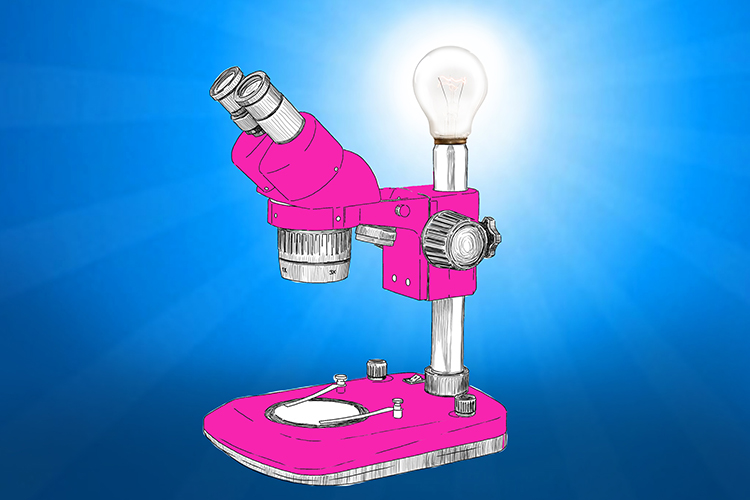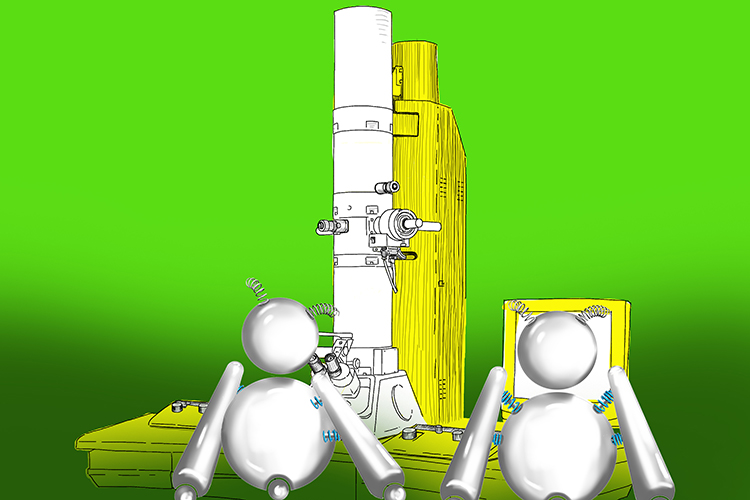# Calculating magnification and sizes of specimens

Microscopes are used to study the cells of living things.

A light microscope – which uses visible light – can magnify images up to about 1,500 times actual size.The microscope had a really bright light on it (light microscope).

An electron microscope uses electron beams in place of light and can magnify up to around two million times.The electronic robots (electrons) check out the images provided by the electron microscope.

The magnification of a specimen can be calculated using the following equation:

magnification=\frac{measured\ size}{actual\ size}

Example:

A plant cell in the microscope image measures 12mm across. The actual size of the cell is 0.012mm. So, in this instance:

magnification\ =\frac{12}{0.012}

Which means the magnification is 1000 times.

The actual size of a biological specimen can be calculated using this equation:

actual\ size=\frac{measured\ size}{magnification}

Example:

The nucleus of a cell in a magnified image is 4mm across. The magnification of the image is 800 times, so in this case:

actual\ size\ =\frac{4}{800}

Which means the actual size is 0.005mm.

NOTE:

Actual sizes of specimens or parts of specimens are often measured in micrometres. You just need to remember that a micrometre is 1000th of a millimetre (or a millionth of a metre).

So, an actual size of 0.012mm would be 12 micrometres (0.012 X 1000), and an actual size of 0.005mm would be 5 micrometres (0.005 X 1000).

If you need to work out the magnification of a specimen and one figure is in micrometres and the other in millimetres, simply convert one measurement to the unit you want your answer to be in.

Example:

Measured size 5mm; actual size 2 micrometres